# Compound of two tetrahedra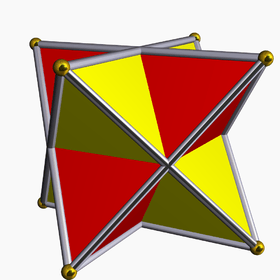In geometry, a compound of two tetrahedra is constructed by two overlapping tetrahedra, usually implied as regular tetrahedra.

There is only one uniform polyhedral compound, the stellated octahedron, which has octahedral symmetry, order 48. It has a regular octahedron core, and shares the same 8 vertices with the cube.

## Lower symmetry constructions

There are lower symmetry variations on this compound, based on lower symmetry forms of the tetrahedron.

Examples
D4h, [4,2], order 16 D4, , order 8 D3d, [2+,6], order 12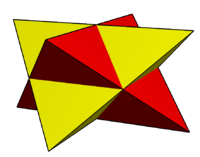Compound of two tetragonal disphenoids in square prism
ß{2,4} or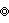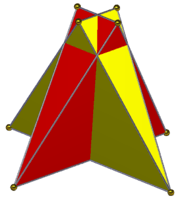Compound of two digonal disphenoids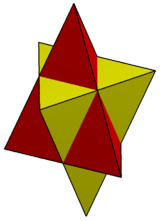Compound of two
right triangular pyramids in triangular trapezohedron

## Other compounds

If two regular tetrahedra are given the same orientation on the 3-fold axis, a different compound is made, with D3h, [3,2] symmetry, order 12.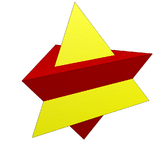Other orientations can be chosen as 2 tetrahedra within the compound of five tetrahedra and compound of ten tetrahedra: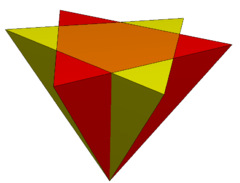## References

• Cundy, H. and Rollett, A. Five Tetrahedra in a Dodecahedron. §3.10.8 in Mathematical Models, 3rd ed. Stradbroke, England: Tarquin Pub., pp. 139-141, 1989.